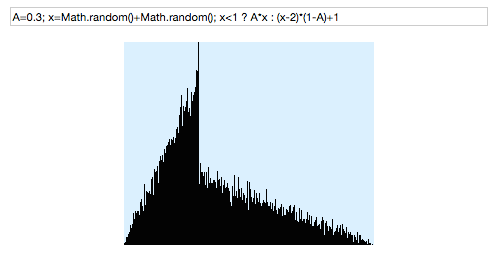Posted by: pixelero | November 13, 2014

## “How do I generate weighted random numbers?”

Hi there folks!

It looks like one link leading most visitors to this blog is a discussion on a programming forum and that this old post of mine about random numbers is among the most popular ones. No wonder, there’s not much information about how to modify the distribution of random variables easily available.
In this case the basic question is how to pick random variables on a certain range 0…N so that the main weight is one a desired value A in that range e.g. Meaning that if A is near zero, the expected value is small, and vice versa large when A approaches N.

Solution 1:
First thing that comes to mind is that taking the maximum of two Math.randoms returns a result near 1.0, and likewise the minimum remains near zero.Now if we take an linear average of these two with respect to the desired center value A we get a formula:
A=0.3;(1-A)*Math.min(Math.random(),Math.random()) + A*Math.max(Math.random(),Math.random())
Here’s the result of that with A=0.3 on my old demo:Ok, looks like that pretty close to what was asked, although four Math.randoms are needed for one single result. What would this look like a mathematical formula? Well, I’m not going to calculate it but according to probabilistic theories the sum of two distributions is their convolution.
Just try it with different values for A in range 0…1.
Another solution with a more Gaussian form would be like for instance A+(Math.random()+Math.random()-Math.random()-Math.random())/4 although you have to remove the values outside of the range 0 to 1, see also Irwin-Hall distribution.

Solution 2:
Then again, could we possible find a more exact solution for this problem? Let’s now have a look on an analytic solution, how to adapt a random variable to a chosen distribution by a mathematical formula.
First we need a function y=f(x) for the wished probability density. In this case it’s a piecewise defined function: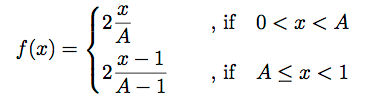The factor of 2 comes from the fact that the sum of all probabilities, when integrated over 0<x<1 must be equal to 1.0. The integral function of this, aka Cumulative distribution function F(x) or CDF tells us the probability of a random variable is below x: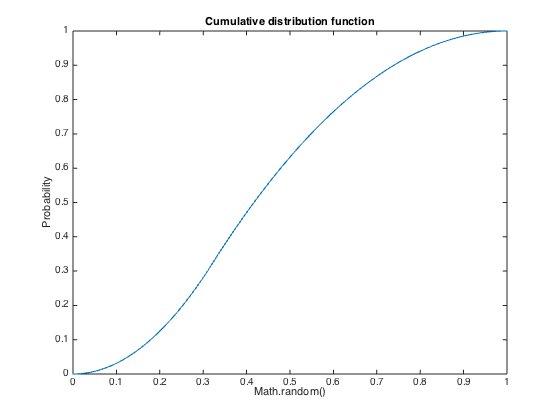The function we were actually looking for, is the inverse of the F(x). In the process of finding out this result, there are two phases which might prove hard to solve, first the integration and then solving the inverse, but now we turned lucky. This is function we’ll apply to a random number to get the distribution we were after: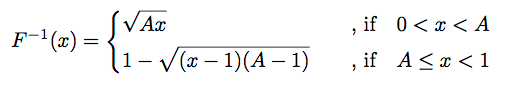Now looking at that formula, who would have guessed this is the result?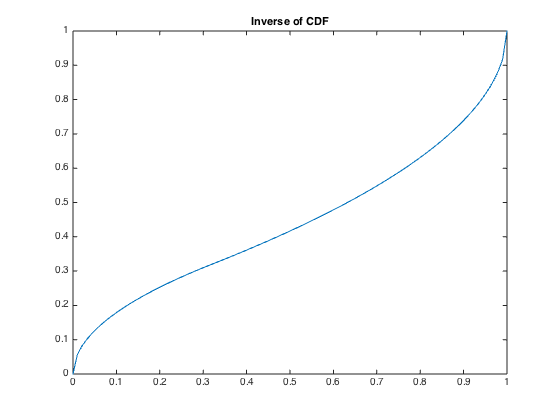Now writing that as a piece of javascript code would be:
A=0.3;x=Math.random(); x<A ? Math.sqrt(A*x) : 1-Math.sqrt((A-1)*(x-1));Comparing it to the first result shows that this has a smoother more obviously linear distribution around value A.

Solution 3:
In both these solutions the probability is at highest at x=A, but the mean of the distribution might not be E(x)=∫x p(x) dx=A.
Let’s choose some distribution with E(x)=A as a starting point, one such would be
A=0.3; x=Math.random(); x<0.5 ? 2*A*x : 2*(x-1)*(1-A)+1That works so that values below x=0.5 are mapped to range 0.0…A and likewise values x>0.5 to range A…1.0: half the probability is now below A and the other half above A. But the distribution on both sides is uniform, and we want the highest probability to X=A?
Now averaging two similar samples doesn’t change the mean value, but puts the highest probability to x=A, and the result is:
A=0.3; x=Math.random()+Math.random(); x<1.0 ? A*x : (x-2)*(1-A)+1